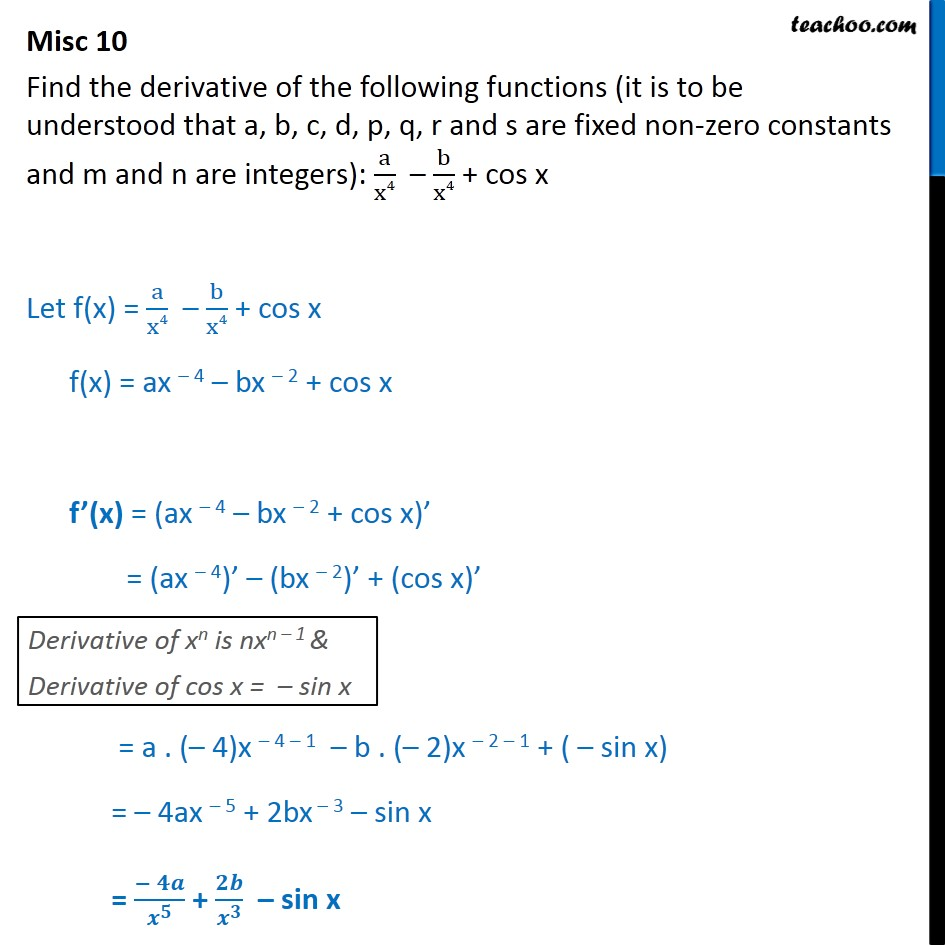Miscellaneous

Chapter 12 Class 11 Limits and Derivatives
Serial order wiseLearn in your speed, with individual attention - Teachoo Maths 1-on-1 Class

### Transcript

Misc 10 Find the derivative of the following functions (it is to be understood that a, b, c, d, p, q, r and s are fixed non-zero constants and m and n are integers): a x4 b x4 + cos x Let f(x) = a x4 b x4 + cos x f(x) = ax 4 bx 2 + cos x f (x) = (ax 4 bx 2 + cos x) = (ax 4) (bx 2) + (cos x) = a . ( 4)x 4 1 b . ( 2)x 2 1 + ( sin x) = 4ax 5 + 2bx 3 sin x = + sin x